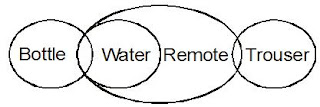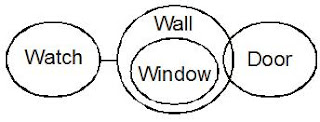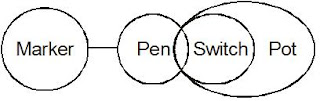# Reasoning Ability || 06 – 09 – 2018

Dear Aspirants,
Welcome to Mentor for Bank Exams. Here is the Reasoning Ability Quiz to help you practice with the best of latest pattern questions for the upcoming IBPS PO, IBPS Clerk and other bank and Insurance Exams.
Directions (1 – 5): In each question given below three statements are followed by two conclusions numbered I and II. You have to take the three given statements to be true even if they seem to be at variance from the commonly known facts. Read the conclusions and decide which logically follows from the three given statements disregarding commonly known facts :
1. Statements :  Some remotes are trousers. Some water are bottles. All water are remotes.
Conclusions: (I)    Some remotes are bottles.  (II)  All trousers are bottles.
a) If only conclusion I follows.
b) If only conclusion II follows.
c) If either conclusion I or II follows.
d) If neither conclusion I nor II follows.
e) If both conclusion I and II follow.
Solution:2. Statement :  All papers are books. Some copies are books. All copies are pens.
Conclusion : (I)  All books are copies is being a possibility. (II) No pen is paper.
a) If only conclusion I follows.
b) If only conclusion II follows.
c) If either conclusion I or II follows.
d) If neither conclusion I nor II follows.
e) If both conclusion I and II follow.
Solution:3. Statement : No watch is wall. All windows are walls.  Some doors are walls.
Conclusion : (I) Some windows are watches.
(II)  Some doors are windows
a) If only conclusion I follows.
b) If only conclusion II follows.
c) If either conclusion I or II follows.
d) If neither conclusion I nor II follows.
e) If both conclusion I and II follow.
Solution:4. Statement : Some tables are chairs.      No wood is table.        All woods are fans.
Conclusions :
(I)   All fans are tables.
(II)  Some chairs being wood is a possibility.
a) If only conclusion I follows.
b) If only conclusion II follows.
c) If either conclusion I or II follows.
d) If neither conclusion I nor II follows.
e) If both conclusion I and II follow.
Solution:5. Statement : No pen is marker.       Some switches are pens.  All switches are pots.
Conclusions :
(I)  Some pens are pots.
(II) Some switches are markers.
a) If only conclusion I follows.
b) If only conclusion II follows.
c) If either conclusion I or II follows.
d) If neither conclusion I nor II follows.
e) If both conclusion I and II follow.
Solution:Directions (6 – 10): Study the following information carefully and answer the question asked:-
Twelve people are sitting in two parallel rows containing six people each in such a way that there is equal distance between adjacent persons. In row 1, J, K, L, M, N and O are seated and all of them are facing South. In row 2, A, B, C, D, E and F are seated and all of them are facing North. Therefore in the given seating arrangement each member seated in a row faces another member of the other row, A sits third to the right of D. Neither D nor A sits at any of the extreme end. Only two people sit between B and E. Two persons sit between K and L. E does not face O. N faces D. O does not sit at any of the extreme ends. B sits at the extreme ends. L is not an immediate neighbour of N. J is not an immediate neighbour of L. C does not face O.

6. Who among the following sit at the extreme ends of the row?
a) C, A
b) J, L
c) M, N
d) B, D
e) C, F

7. Who among the following faces A?
a) N
b) J
c) O
d) M
e) None of these

8. How many person are there between M and N?
a) One
b) None
c) Two
d) Three
e) None of these

9. How many person are there between M and N?
a) One
b) None
c) Two
d) Three
e) None of these